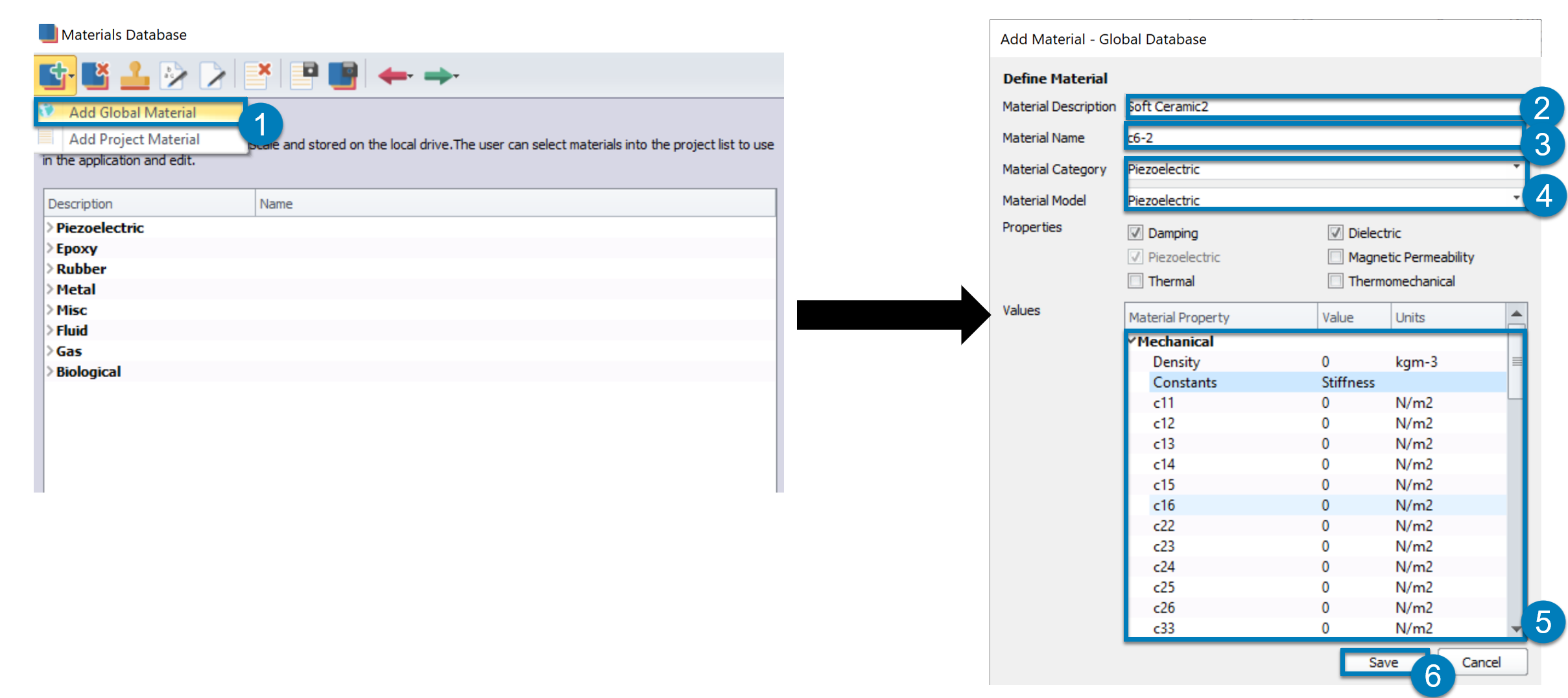# Section 1: Introduction

## Example of Material Datasheet

• Obtaining the correct piezoelectric material properties from a manufacturer datasheet and transforming those properties into the correct format can be a hassle.
• In this article, I will use the datasheet on the right here and I will convert the soft ceramic material "C-6" present on this datasheet into a format which can be inserted into the material database of OnScale.
• Note that if the image is to small, you can click on it to make it bigger.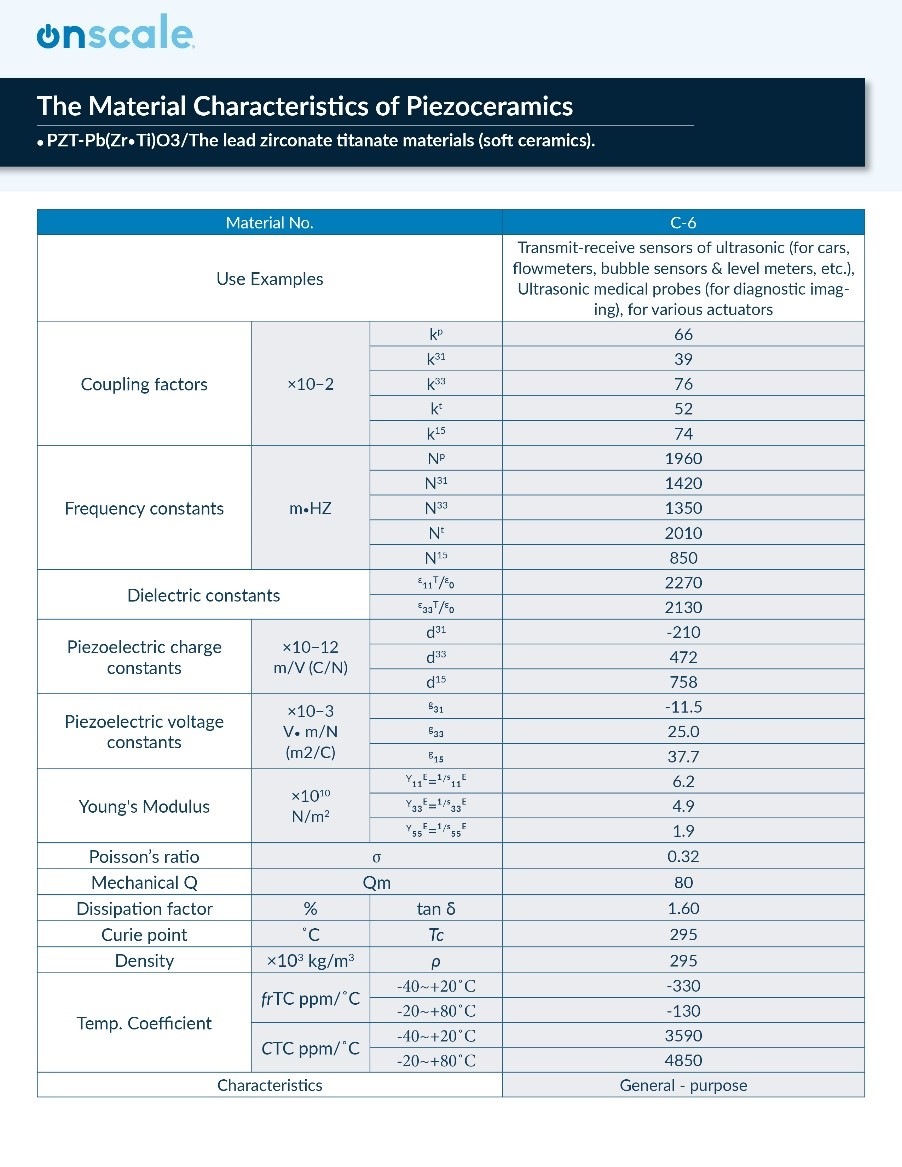Let's see together how to do that.

But first, we need to look at some of the equations defining piezoelectricity to understand more closely what those material coefficients mean.

## Quick Reminder about the Piezoelectric Effect

• The piezoelectric effect results from the linear electromechanical interaction between the mechanical and electrical states in crystalline materials
• The piezoelectric effect is a reversible process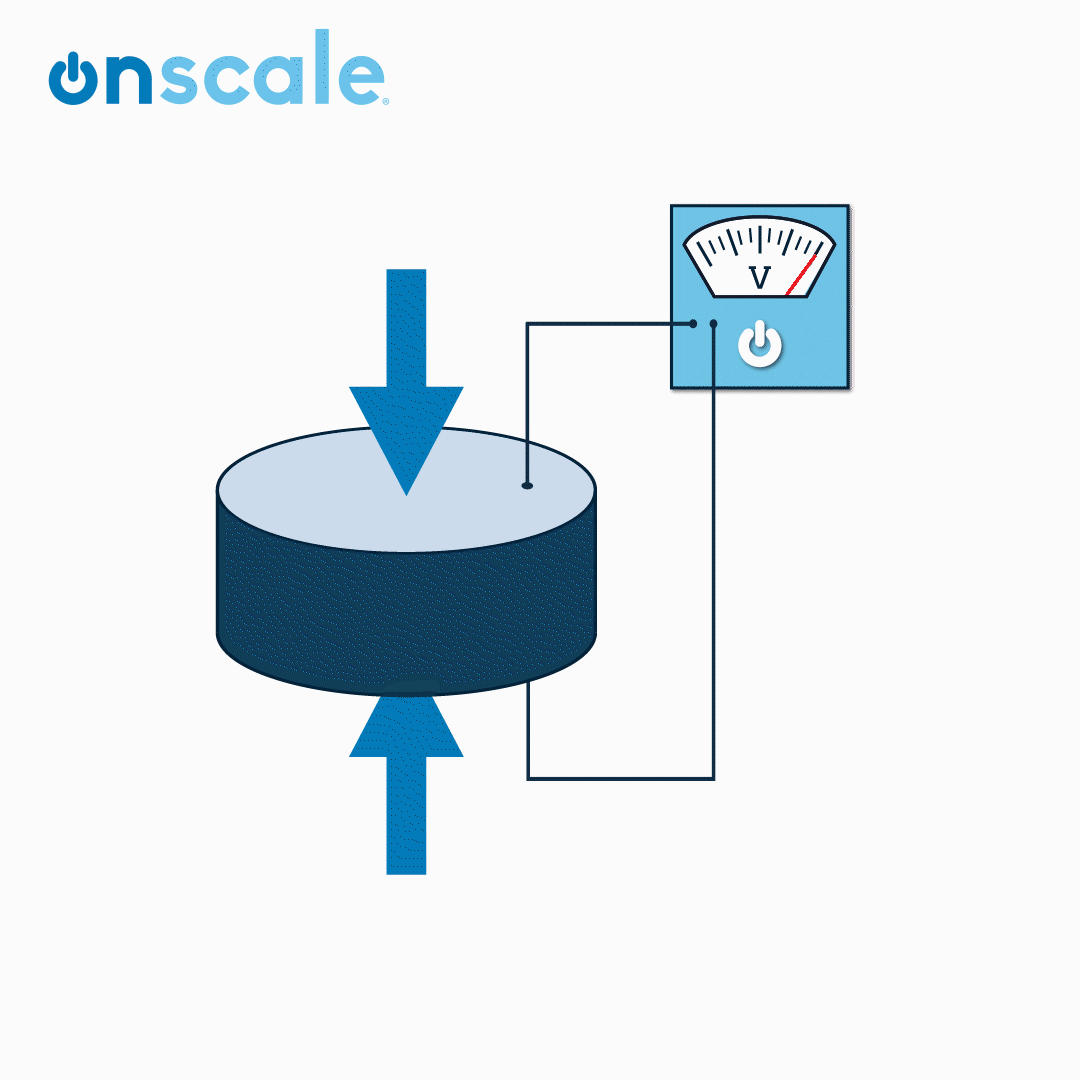## The Equations of Piezoelectricity

Linear piezoelectricity is the combined effect of electrical and elastic mechanical behavior. Those behaviors are defined by the following two laws.

The linear electrical behavior of the material: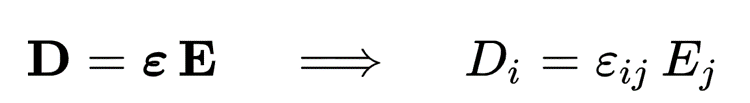D is the electric charge density displacement (electric displacement)

ε is permittivity (free-body dielectric constant)

E is electric field strength

The Hooke’s law for elastic materials: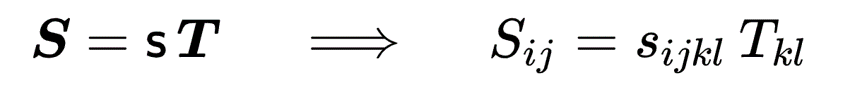S is strain

s is compliance under short-circuit conditions

T is stress

These relations may be combined into so-called coupled equations, of which the strain-charge form is: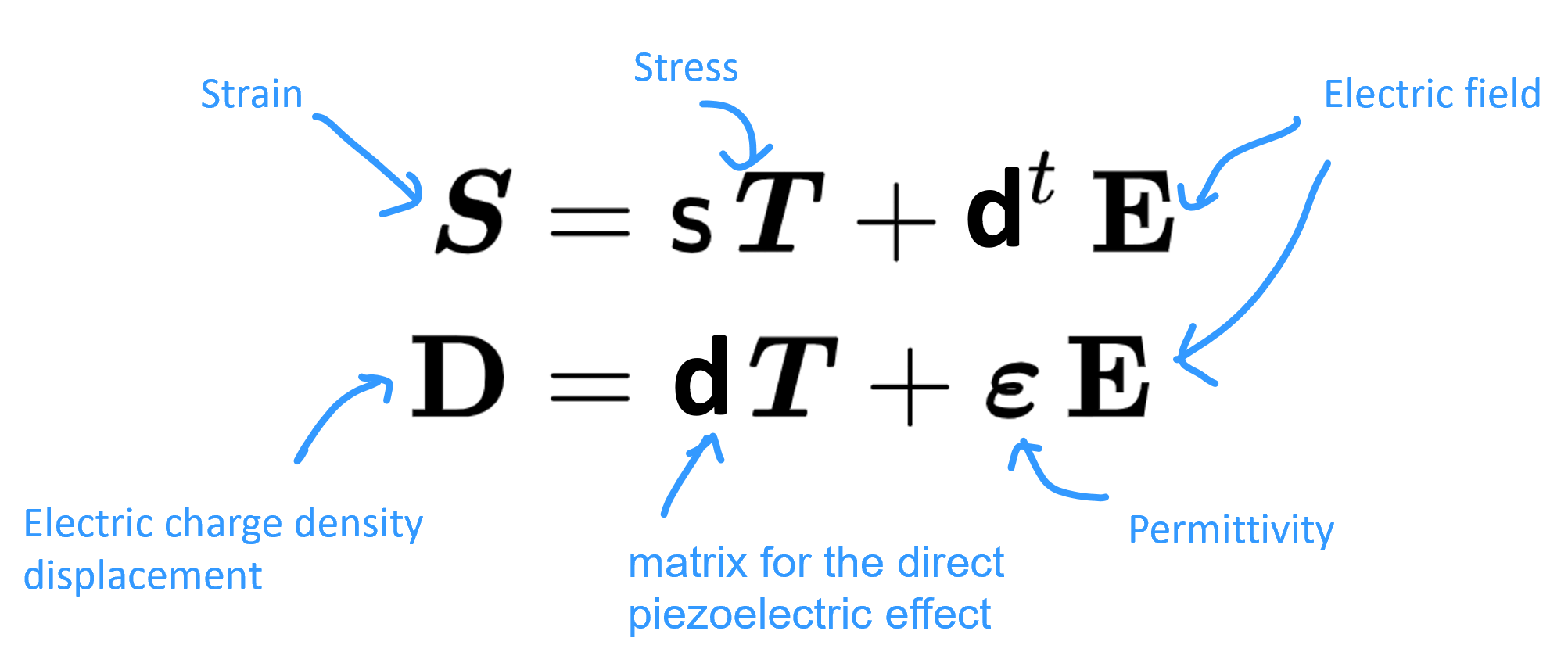Of course, we need to think about those equations in terms of their matrix equivalent.

## Strain-charge Matrix relation for the tetragonal PZT C4V

For example, The strain-charge for a material of the 4mm (C4v) crystal class (such as a poled piezoelectric ceramic such as tetragonal PZT or BaTiO3) as well as the 6mm crystal class may also be written as (ANSI IEEE 176):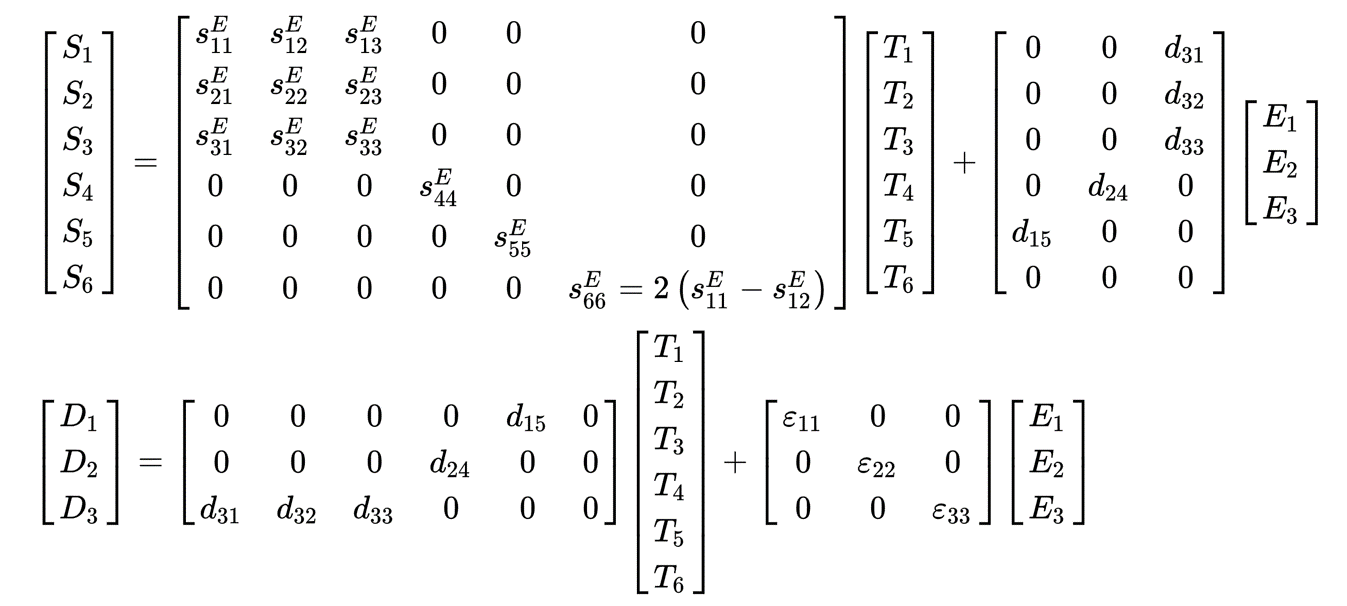## The 4 Constitutive Piezoelectric equations

In practice, piezoelectric coefficients can be defined in four ways as follows: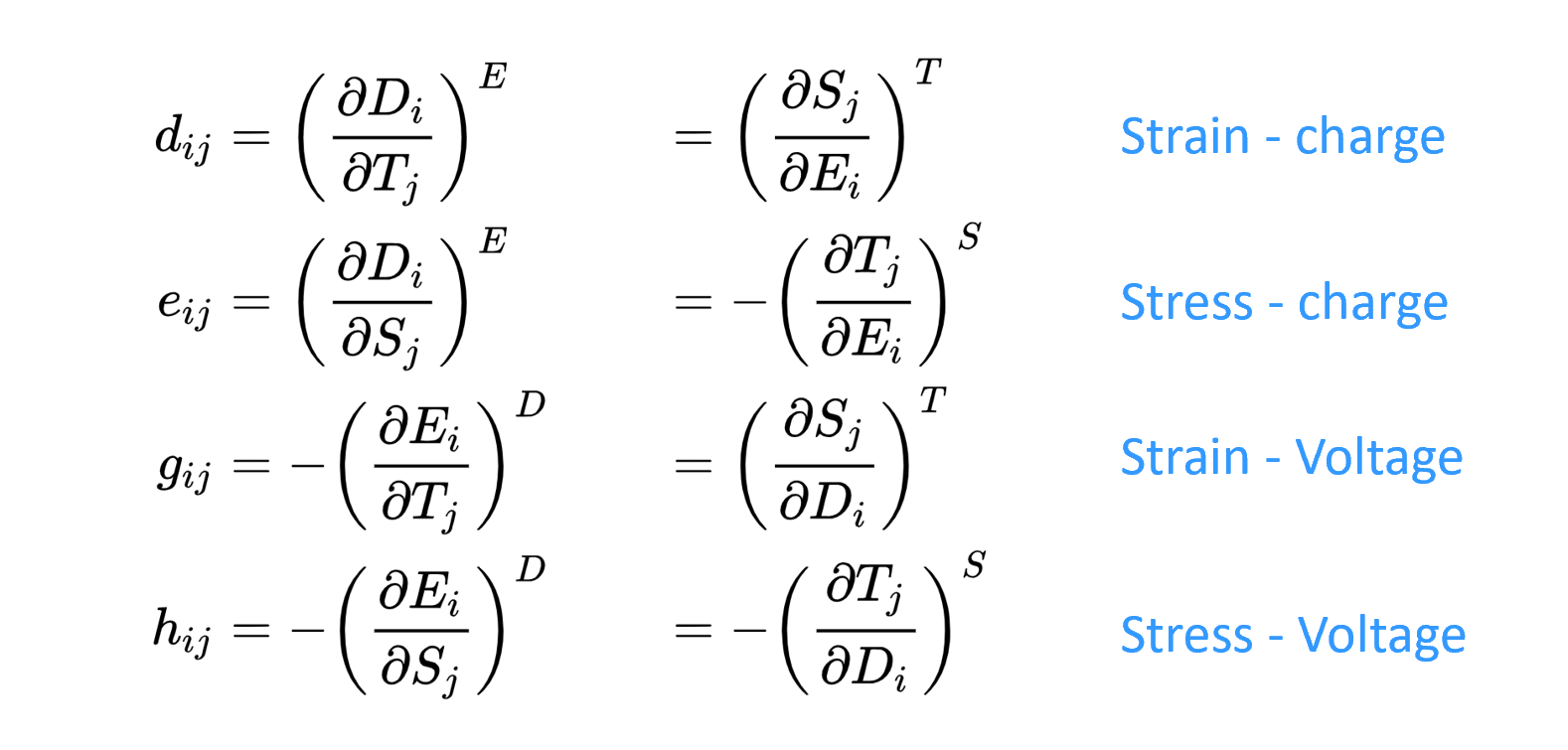And because of that, we can define the previous 2 equations in 4 different "formats" in function of the coefficients we want to use: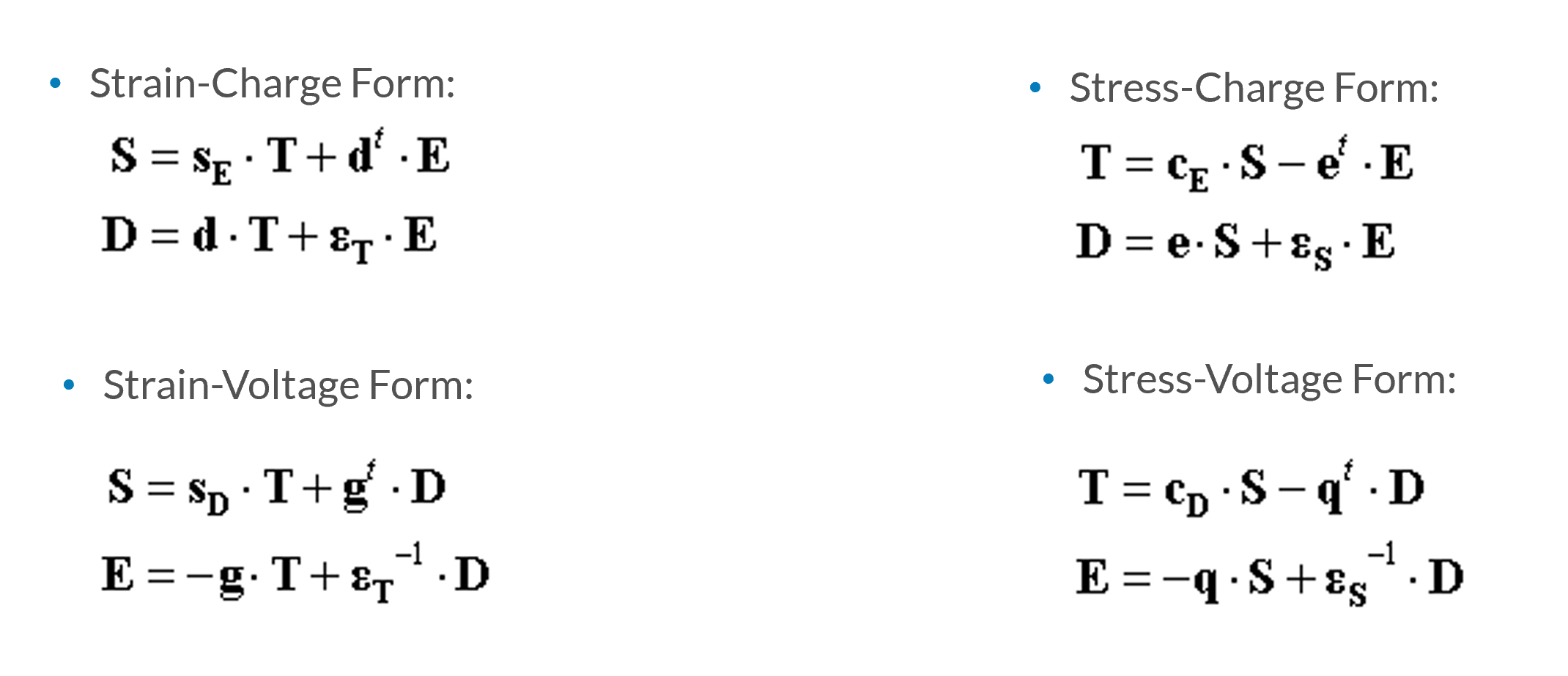# Section 2: What do we need in OnScale to define a new piezoelectric material?

The accurate modelling of piezoelectric materials requires 13 independent parameters:

Note: In OnScale, you can input directly the relative permittivities εxx and εzz. The matrix forms [εT] or [εS] can be calculated from εxx and εzz in case they are required to perform certain transformations.

If we look at the material properties in OnScale for a material such as pmt3, that translates like this: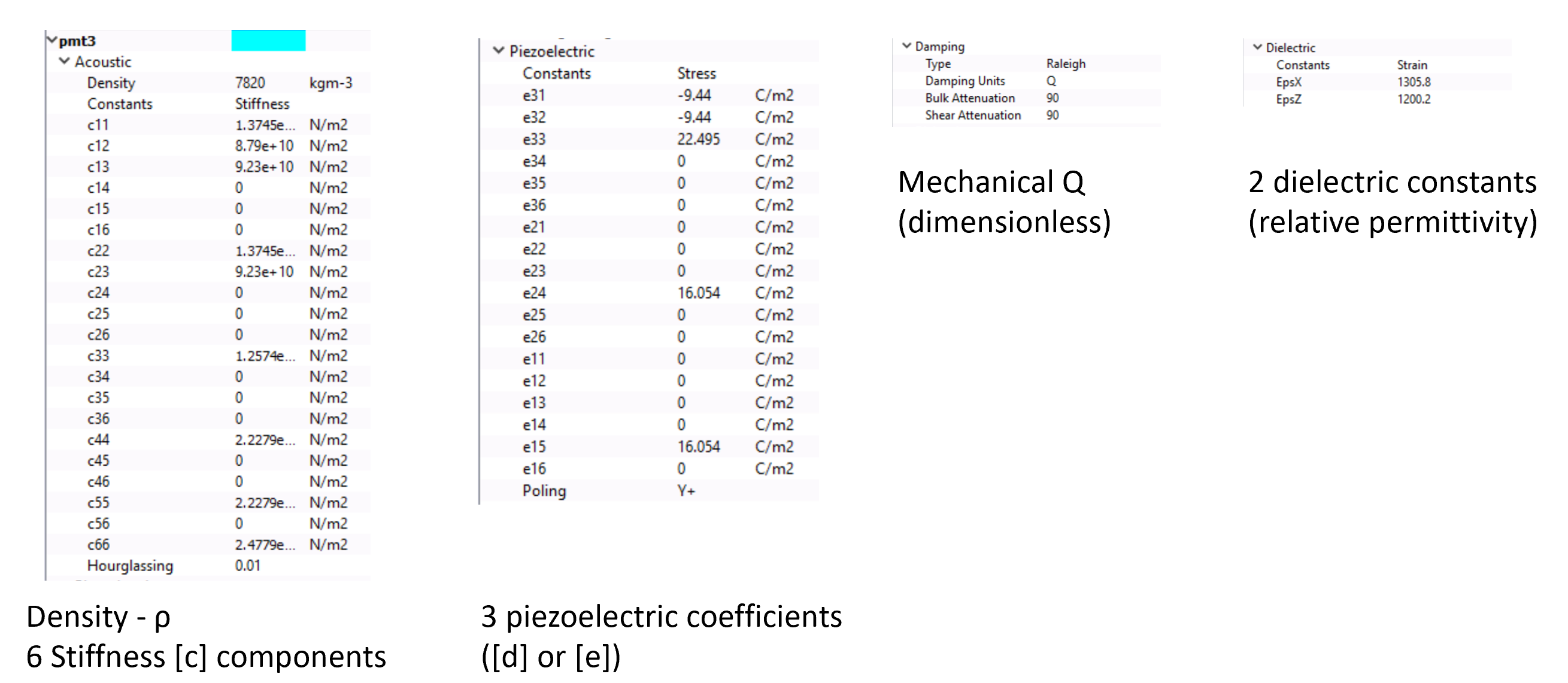## Which parameter do I have already on the material datasheet and which parameter should I calculate?

For this material datasheet, we see that we got the density, the mechanical Q and the 2 dielectric constants (also called relative permittivity).

We will need to calculate the stiffness matrix (1) using the young's modulus and the poisson's ratio provided. Then, we will need to calculate the matrix [e] (2) of piezoelectric coefficients from the matrix [d] provided.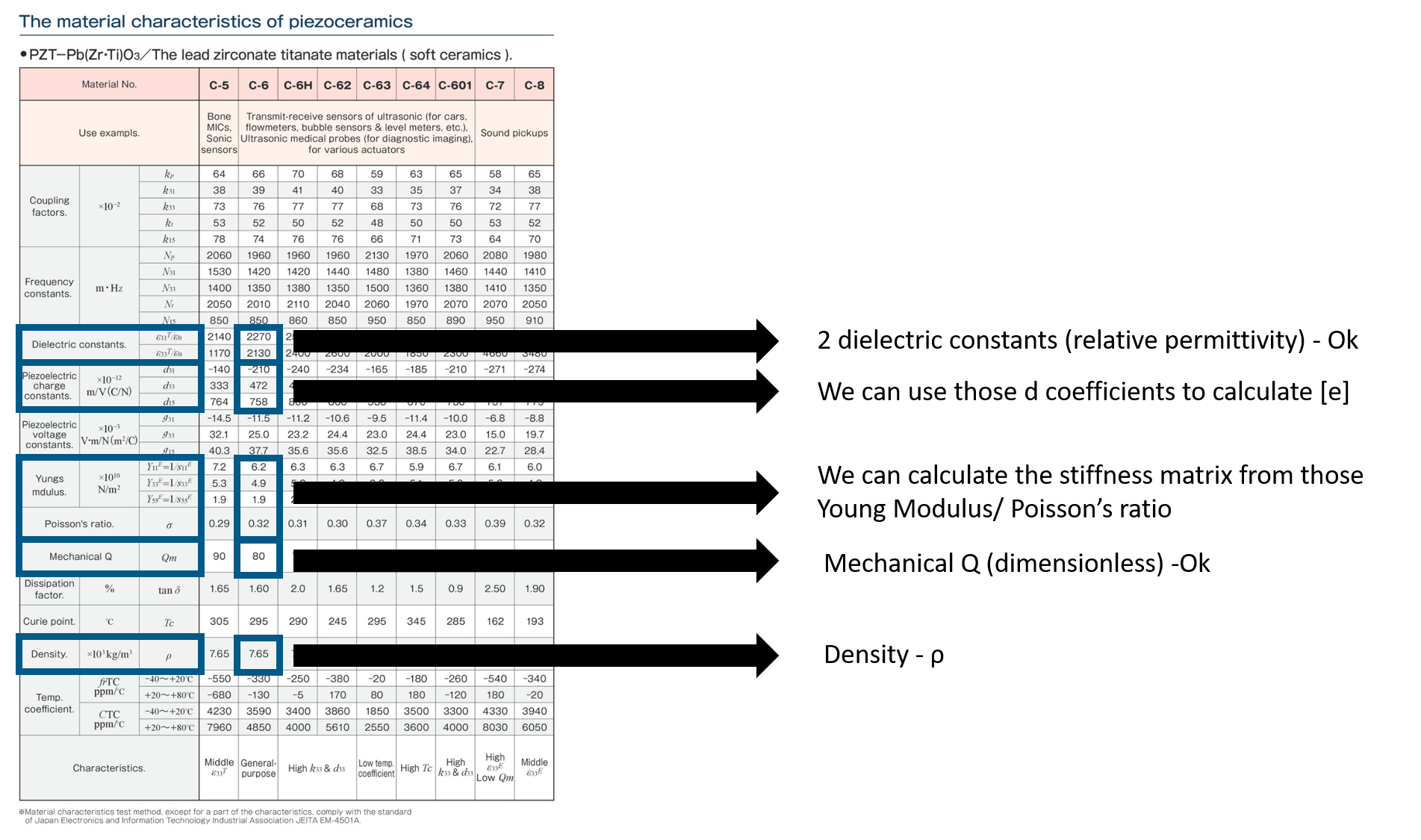## 1- Calculation of the stiffness matrix

We need first to calculate the compliance matrix and then we can inverse it to obtain the stiffness matrix. As PZT materials exhibits transverse isotropy (6mm crystal symmetry), we can use the following matrix to calculate the compliance matrix: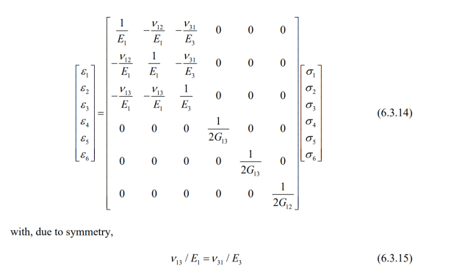The transverse modulus G12 is related to the transverse Poisson's ratio and the transverse stiffness through the following equation: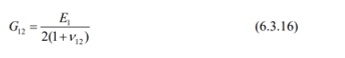Here's a python script that you can use to calculate this compliance matrix and the associated stiffness matrix (which is the inverse of the compliance matrix).

```import numpy as np
from numpy.linalg import inv

E1 = 6.2e10 #N/m2
E2 = 6.2e10 #took E2=E1
E3 = 4.9e10
nu = 0.32
E5 = 1.9e10
G6 = E1/(2*(1+nu))

#compliance matrix
s = np.array([ [1/E1,-nu/E2,-nu/E3,0,0,0], [-nu/E2,1/E2,-nu/E3,0,0,0], [-nu/E3,-nu/E3,1/E3,0,0,0], [0,0,0,1/(2*E5),0,0], [0,0,0,0,1/(2*E5),0], [0,0,0,0,0,1/G6]])

#print (s)

#stiffness matrix
c = inv(s)

for i in range(6):
for j in range(6):
print('c'+str(i+1)+str(j+1)+'='+str(c[i][j]))
```

Note: To run this script, you will need to install Python first and then the module numpy of python.

This is what you should obtain:

Compliance Matrix

```[[ 1.61290323e-11 -5.16129032e-12 -6.53061224e-12  0.00000000e+00  0.00000000e+00  0.00000000e+00]
[-5.16129032e-12  1.61290323e-11 -6.53061224e-12  0.00000000e+00  0.00000000e+00  0.00000000e+00]
[-6.53061224e-12 -6.53061224e-12  2.04081633e-11  0.00000000e+00   0.00000000e+00  0.00000000e+00]
[ 0.00000000e+00  0.00000000e+00  0.00000000e+00  2.63157895e-11  0.00000000e+00  0.00000000e+00]
[ 0.00000000e+00  0.00000000e+00  0.00000000e+00  0.00000000e+00  2.63157895e-11  0.00000000e+00]
[ 0.00000000e+00  0.00000000e+00  0.00000000e+00  0.00000000e+00  0.00000000e+00  4.25806451e-11]]
```

Stiffness Matrix

```[[9.71426186e+10 5.01729217e+10 4.71409729e+10 0.00000000e+00  0.00000000e+00 0.00000000e+00]
[5.01729217e+10 9.71426186e+10 4.17508418e+10 0.00000000e+00  0.00000000e+00 0.00000000e+00]
[4.71409729e+10 4.71409729e+10 7.91702226e+10 0.00000000e+00  0.00000000e+00 0.00000000e+00]
[0.00000000e+00 0.00000000e+00 0.00000000e+00 3.80000000e+10  0.00000000e+00 0.00000000e+00]
[0.00000000e+00 0.00000000e+00 0.00000000e+00 0.00000000e+00  3.80000000e+10 0.00000000e+00]
[0.00000000e+00 0.00000000e+00 0.00000000e+00 0.00000000e+00  0.00000000e+00 2.34848484e+10]]
```

## 2- Calculation of Piezoelectric [e] coefficients from [d]

Now that we have the Stiffness Matrix, we can calculate the coefficient [e] from the coefficient [d] written on the material datasheet.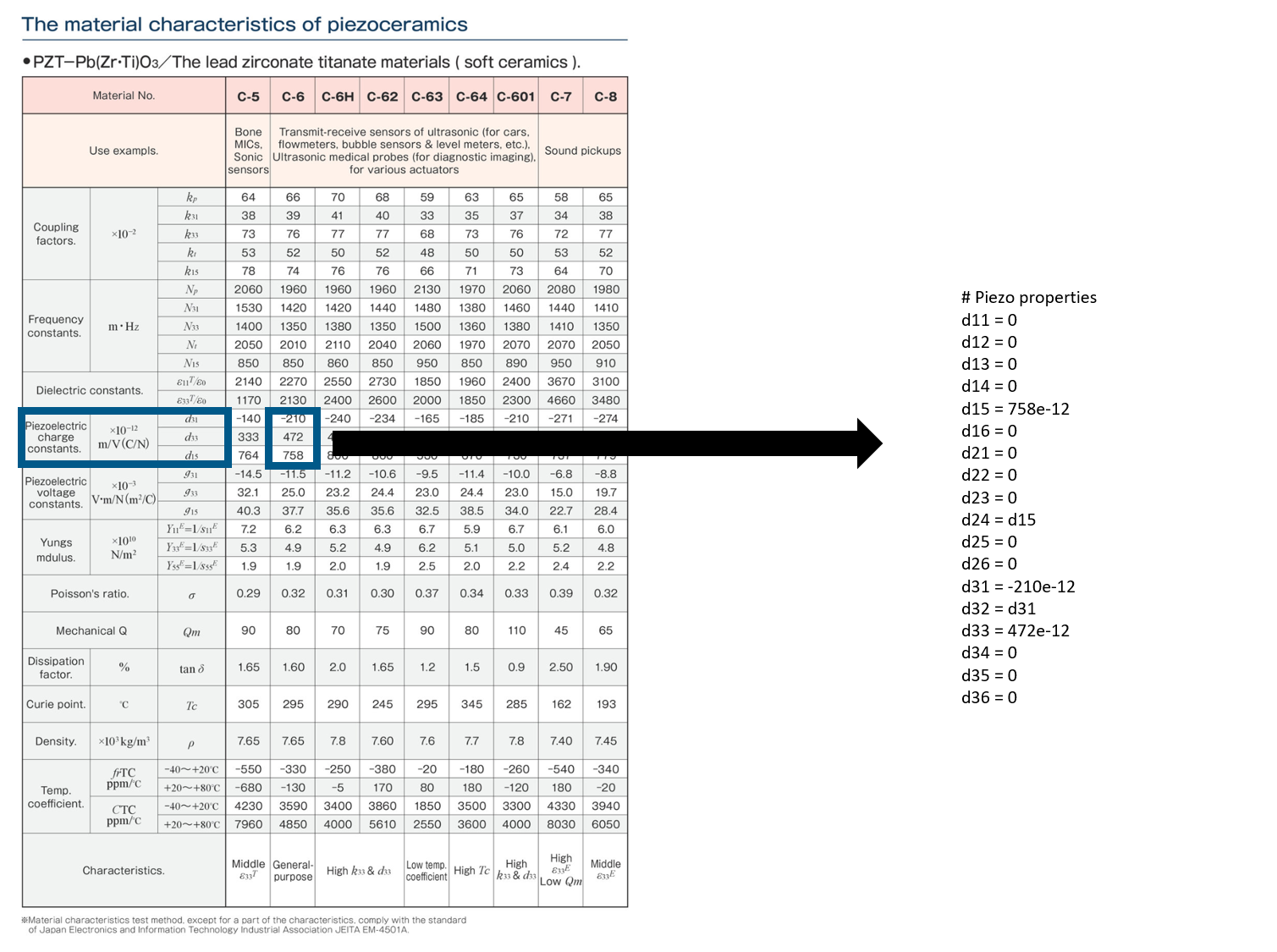To transform those [d] coefficients, we need to have a look at some of the transformation relations mentioned on this page from the efunda website:

Stress-Charge to Strain-Charge: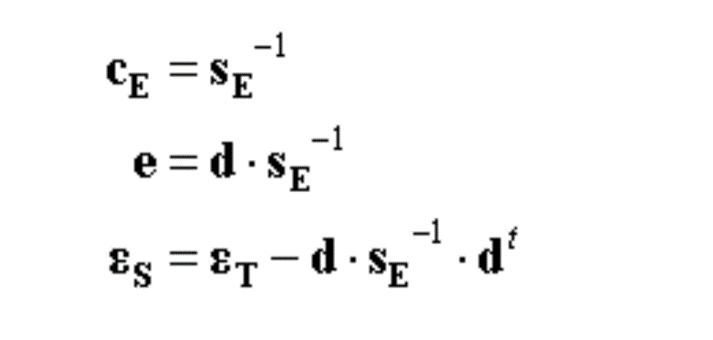This tells us the following:

• To calculate [e], we need to use the relation [e] = [d].[c]
• [c] is the stiffness matrix calculated previously

Note: [c] is the stiffness matrix, NOT the compliance matrix, it's a bit confusing

I can now use the following Python script to calculate:

```import numpy as np
from numpy.linalg import inv

# Piezo properties
d11 = 0
d12 = 0
d13 = 0
d14 = 0
d15 = 758e-12
d16 = 0
d21 = 0
d22 = 0
d23 = 0
d24 = d15
d25 = 0
d26 = 0
d31 = -210e-12
d32 = d31
d33 = 472e-12
d34 = 0
d35 = 0
d36 = 0

d = np.array([[d11,d12,d13,d14,d15,d16],[d21,d22,d23,d24,d25,d26],[d31,d32,d33,d34,d35,d36]])

e = d @ c

for i in range(3):
for j in range(6):
print('e'+str(i+1)+str(j+1)+'='+str(e[i][j]))
```

Which provides me with the following [e] coefficients

```e11=0.0
e12=0.0
e13=0.0
e14=0.0
e15=28.804
e16=0.0
e21=0.0
e22=0.0
e23=0.0
e24=28.804
e25=0.0
e26=0.0
e31=-8.68572426099775
e32=-8.685724260997747
e33=17.569136472961443
e34=0.0
e35=0.0
e36=0.0
```

Now that we have all the coefficients, we are ready to input this new material in OnScale

# Section 3: How to create a new piezo material in OnScale?

To add this new piezoelectric material into the global onscale database, follow the steps below in the material database:

1. Click on Add Global Material